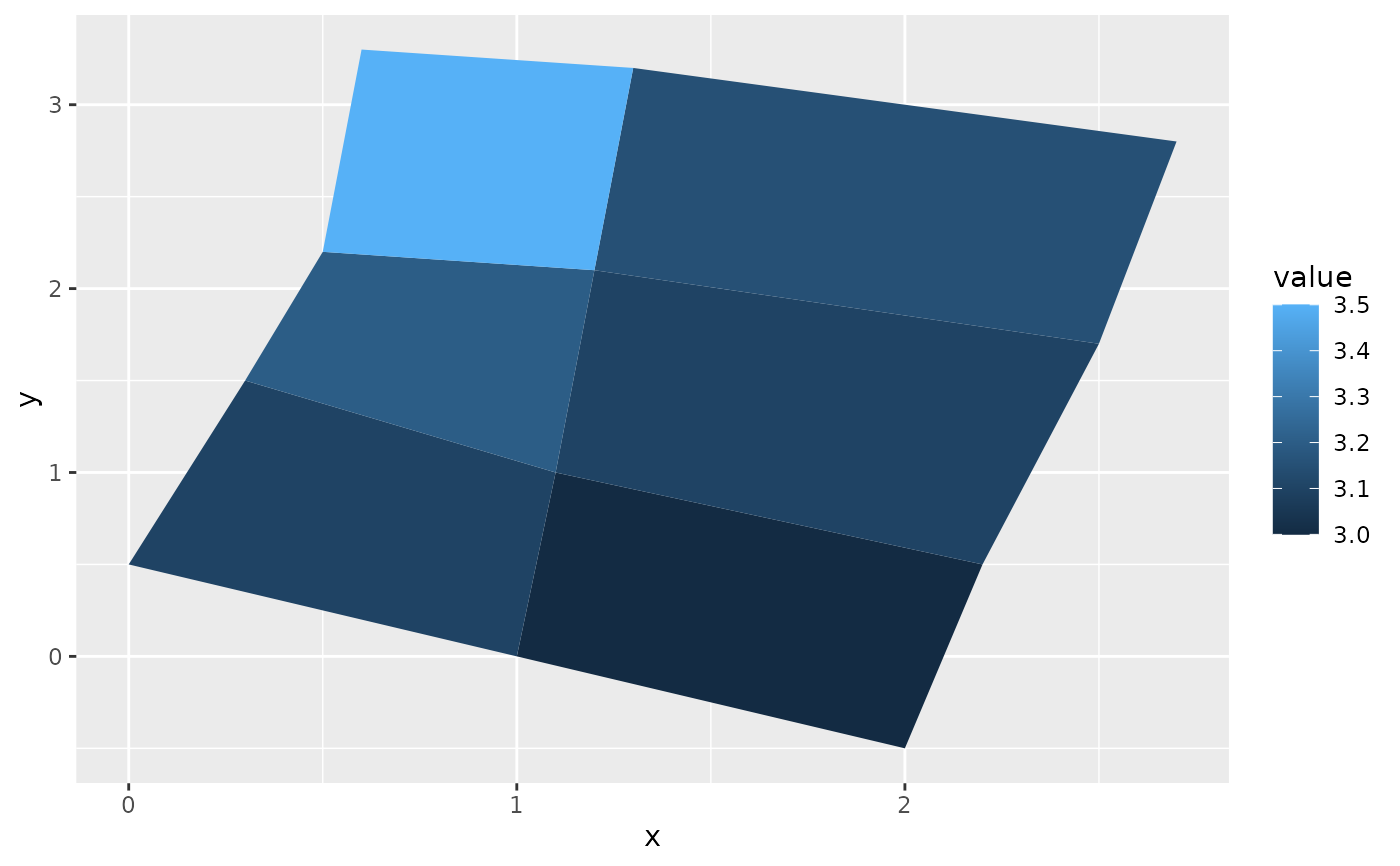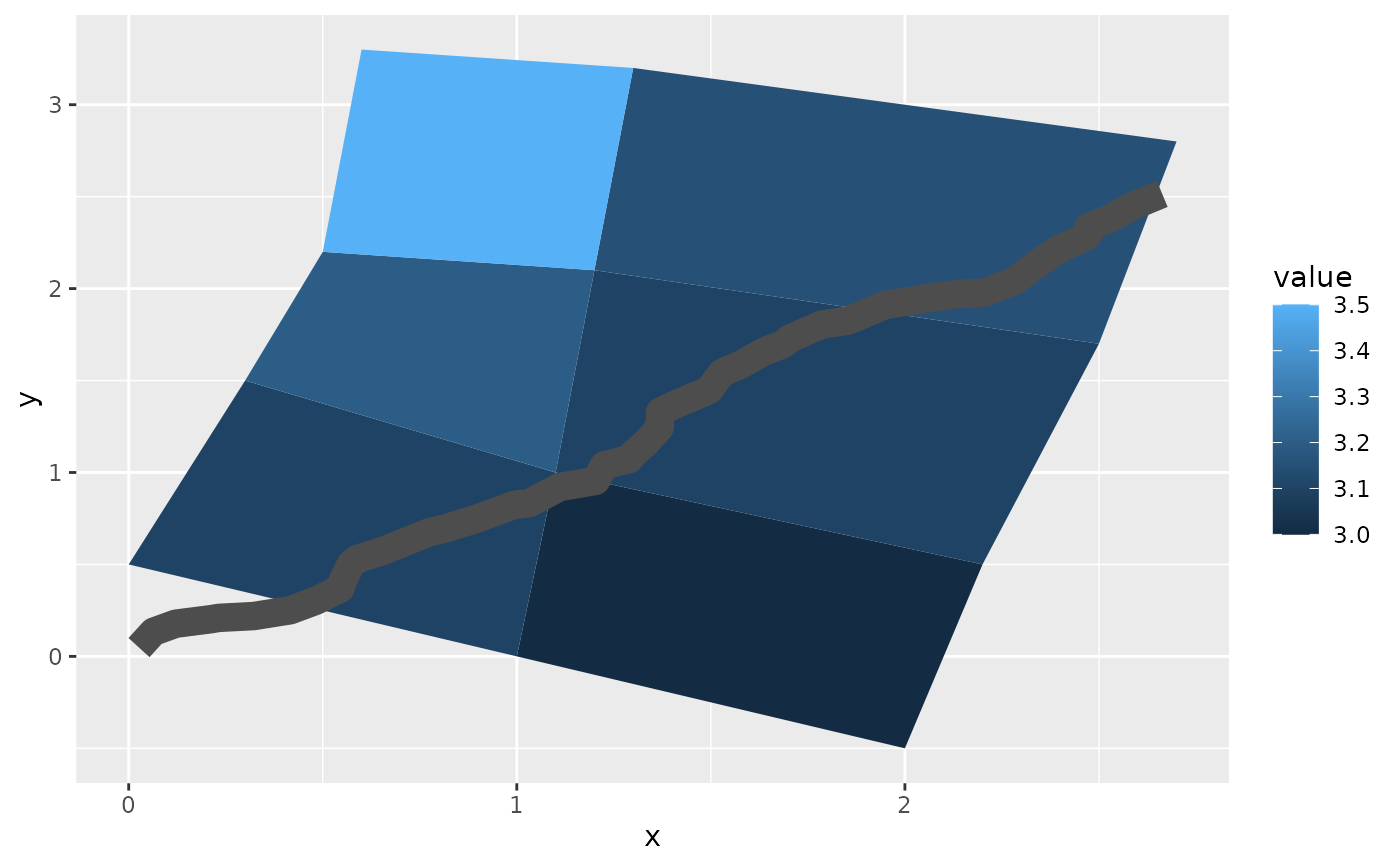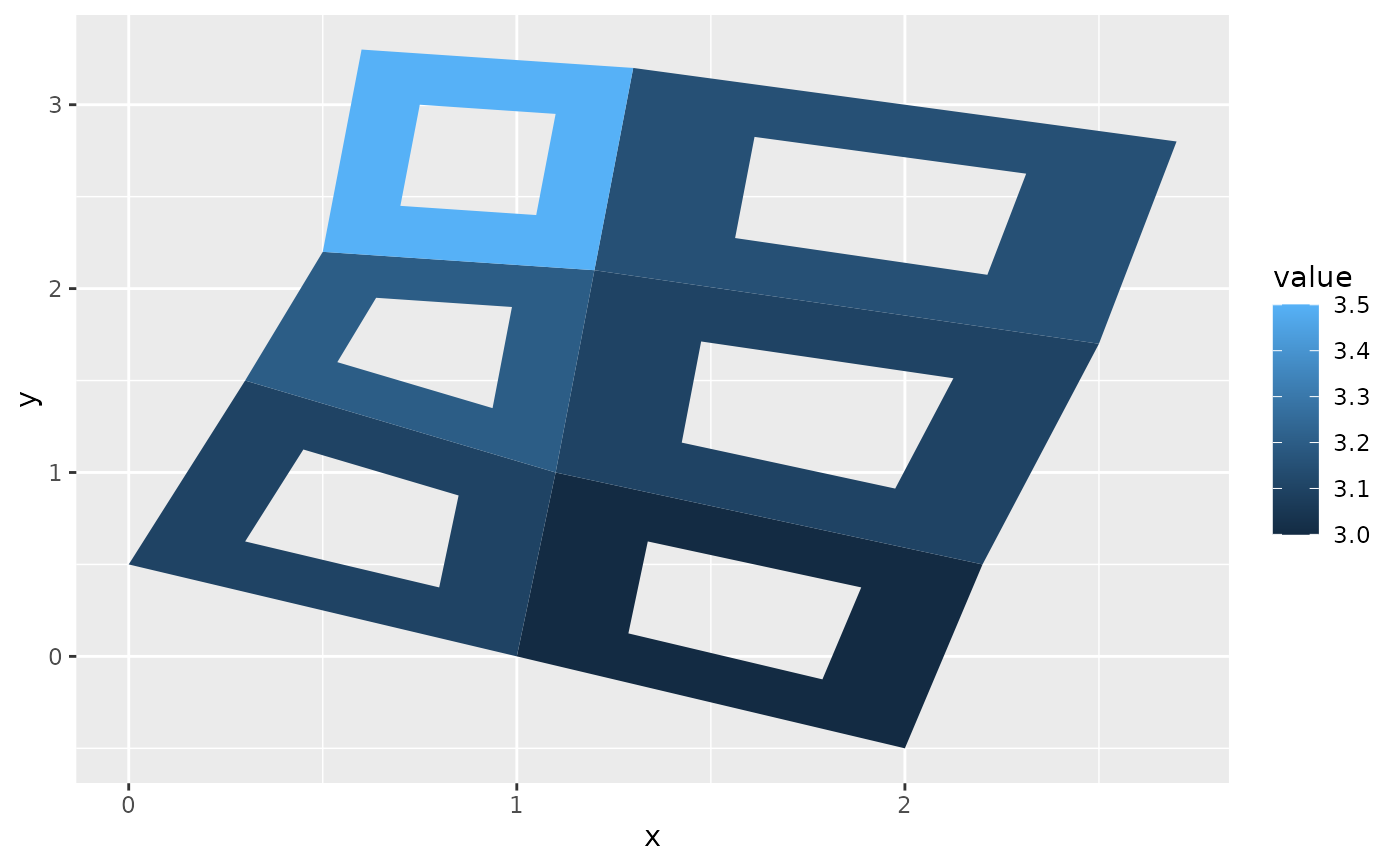Polygons are very similar to paths (as drawn by geom_path()) except that the start and end points are connected and the inside is coloured by fill. The group aesthetic determines which cases are connected together into a polygon. From R 3.6 and onwards it is possible to draw polygons with holes by providing a subgroup aesthetic that differentiates the outer ring points from those describing holes in the polygon.

## Usage

geom_polygon(
mapping = NULL,
data = NULL,
stat = "identity",
position = "identity",
rule = "evenodd",
...,
na.rm = FALSE,
show.legend = NA,
inherit.aes = TRUE
)

## Arguments

mapping

Set of aesthetic mappings created by aes(). If specified and inherit.aes = TRUE (the default), it is combined with the default mapping at the top level of the plot. You must supply mapping if there is no plot mapping.

data

The data to be displayed in this layer. There are three options:

If NULL, the default, the data is inherited from the plot data as specified in the call to ggplot().

A data.frame, or other object, will override the plot data. All objects will be fortified to produce a data frame. See fortify() for which variables will be created.

A function will be called with a single argument, the plot data. The return value must be a data.frame, and will be used as the layer data. A function can be created from a formula (e.g. ~ head(.x, 10)).

stat

The statistical transformation to use on the data for this layer, either as a ggproto Geom subclass or as a string naming the stat stripped of the stat_ prefix (e.g. "count" rather than "stat_count")

position

Position adjustment, either as a string naming the adjustment (e.g. "jitter" to use position_jitter), or the result of a call to a position adjustment function. Use the latter if you need to change the settings of the adjustment.

rule

Either "evenodd" or "winding". If polygons with holes are being drawn (using the subgroup aesthetic) this argument defines how the hole coordinates are interpreted. See the examples in grid::pathGrob() for an explanation.

...

Other arguments passed on to layer(). These are often aesthetics, used to set an aesthetic to a fixed value, like colour = "red" or size = 3. They may also be parameters to the paired geom/stat.

na.rm

If FALSE, the default, missing values are removed with a warning. If TRUE, missing values are silently removed.

show.legend

logical. Should this layer be included in the legends? NA, the default, includes if any aesthetics are mapped. FALSE never includes, and TRUE always includes. It can also be a named logical vector to finely select the aesthetics to display.

inherit.aes

If FALSE, overrides the default aesthetics, rather than combining with them. This is most useful for helper functions that define both data and aesthetics and shouldn't inherit behaviour from the default plot specification, e.g. borders().

## Aesthetics

geom_polygon() understands the following aesthetics (required aesthetics are in bold):

• x

• y

• alpha

• colour

• fill

• group

• linetype

• linewidth

• subgroup

Learn more about setting these aesthetics in vignette("ggplot2-specs").

geom_path() for an unfilled polygon, geom_ribbon() for a polygon anchored on the x-axis

## Examples

# When using geom_polygon, you will typically need two data frames:
# one contains the coordinates of each polygon (positions),  and the
# other the values associated with each polygon (values).  An id
# variable links the two together

ids <- factor(c("1.1", "2.1", "1.2", "2.2", "1.3", "2.3"))

values <- data.frame(
id = ids,
value = c(3, 3.1, 3.1, 3.2, 3.15, 3.5)
)

positions <- data.frame(
id = rep(ids, each = 4),
x = c(2, 1, 1.1, 2.2, 1, 0, 0.3, 1.1, 2.2, 1.1, 1.2, 2.5, 1.1, 0.3,
0.5, 1.2, 2.5, 1.2, 1.3, 2.7, 1.2, 0.5, 0.6, 1.3),
y = c(-0.5, 0, 1, 0.5, 0, 0.5, 1.5, 1, 0.5, 1, 2.1, 1.7, 1, 1.5,
2.2, 2.1, 1.7, 2.1, 3.2, 2.8, 2.1, 2.2, 3.3, 3.2)
)

# Currently we need to manually merge the two together
datapoly <- merge(values, positions, by = c("id"))

p <- ggplot(datapoly, aes(x = x, y = y)) +
geom_polygon(aes(fill = value, group = id))
p# Which seems like a lot of work, but then it's easy to add on
# other features in this coordinate system, e.g.:

set.seed(1)
stream <- data.frame(
x = cumsum(runif(50, max = 0.1)),
y = cumsum(runif(50,max = 0.1))
)

p + geom_line(data = stream, colour = "grey30", linewidth = 5)# And if the positions are in longitude and latitude, you can use
# coord_map to produce different map projections.

if (packageVersion("grid") >= "3.6") {
# As of R version 3.6 geom_polygon() supports polygons with holes
# Use the subgroup aesthetic to differentiate holes from the main polygon

holes <- do.call(rbind, lapply(split(datapoly, datapoly$id), function(df) { df$x <- df$x + 0.5 * (mean(df$x) - df$x) df$y <- df$y + 0.5 * (mean(df$y) - df$y) df })) datapoly$subid <- 1L
holes\$subid <- 2L
datapoly <- rbind(datapoly, holes)

p <- ggplot(datapoly, aes(x = x, y = y)) +
geom_polygon(aes(fill = value, group = id, subgroup = subid))
p
}